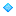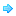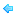EN

NL

EN

简体中文

You will be redirected to our Chinese website.

Contact### Contact

This site works better with javascript enabled.Concentrations of chemicals in the air are usually measured as the mass of chemicals (milligrams, micrograms, nanograms or picograms) per volume of air (cubic meters or cubic feet). Concentrations can also be expressed as parts per million (ppm) or parts per billion (ppb) by using a conversion factor. This conversion factor is based on the molecular weight of the chemical and it is different for every chemical. The temperature of the atmosphere also has an influence on the calculation.

## CONVERT PPM TO MG/M3

In the table below you can easily convert ppm to mg/m3 for diverse gases that may be measured using our air quality monitors.

Formula: concentration (mg/m3) = 0.0409 x concentration (ppm) x molecular weight

For example: 100 ppm of NH3 (17.03 g/mol)
0.0409 x 100 ppm x 17.03 = 69.652Enter the number of mg/m3.
Gas Output Molecular Weight
Ammonia [NH3] M = 17.03 g/mol
Carbon monoxide [CO] M = 28.01 g/mol
Carbon dioxide [CO2] M = 44.01 g/mol
Chlorine [Cl2] M = 70.9 g/mol
Formaldehyde [CH2O] M = 30.026 g/mol
Hydrogen [H2] M = 2.02 g/mol
Methane [CH4] M = 16.04 g/mol
Hydrogen Sulfide [H2S] M = 34.08 g/mol
Nitrogen Dioxide [NO2] M = 46.01 g/mol
Ozone [O3] M = 48.00 g/mol
Perchloroethylene [C2Cl4] M = 165.822 g/mol
Sulfur dioxide [SO2] M = 64.06 g/mol
VOC M = 78.9516 g/mol## CONVERT MG/M3 TO PPM

In the table below you can easily convert ppm to mg/m3 for diverse gases that may be measured using our air quality monitors.

Formula: concentration (ppm) = 24.45 x concentration (mg/m3) ÷ molecular weight

For example: 50 mg/m3 of NH3 (17.03 g/mol)
24.45 x 100 ppm / 17.03 = 71.785ppmEnter the number of mg/m3.
Gas Output Molecular Weight
Ammonia [NH3] M = 17.03 g/mol
Carbon monoxide [CO] M = 28.01 g/mol
Carbon dioxide [CO2] M = 44.01 g/mol
Chlorine [Cl2] M = 70.9 g/mol
Formaldehyde [CH2O] M = 30.026 g/mol
Hydrogen [H2] M = 2.02 g/mol
Methane [CH4] M = 16.04 g/mol
Hydrogen Sulfide [H2S] M = 34.08 g/mol
Nitrogen Dioxide [NO2] M = 46.01 g/mol
Ozone [O3] M = 48.00 g/mol
Perchloroethylene [C2Cl4] M = 165.822 g/mol
Sulfur dioxide [SO2] M = 64.06 g/mol
VOC M = 78.9516 g/molSource: Kansas State University understanding-units-of-measurement.pdf### Are you Interested in measuring gases or particulate matter?

Teesing offers a variety of gas and particulate matter monitoring solutions for indoor and outdoor applications.### ambient air quality monitoring station made affordable

A complete ambient air monitoring station for a wide range of particulate and gaseous pollutants and environmental conditions is available through Teesing.

The AQM’s sensor-based analyzer modules are a unique hybrid between sensors and analyzers with a system designed to be calibrated in situ using Standard Reference Materials for best practice.